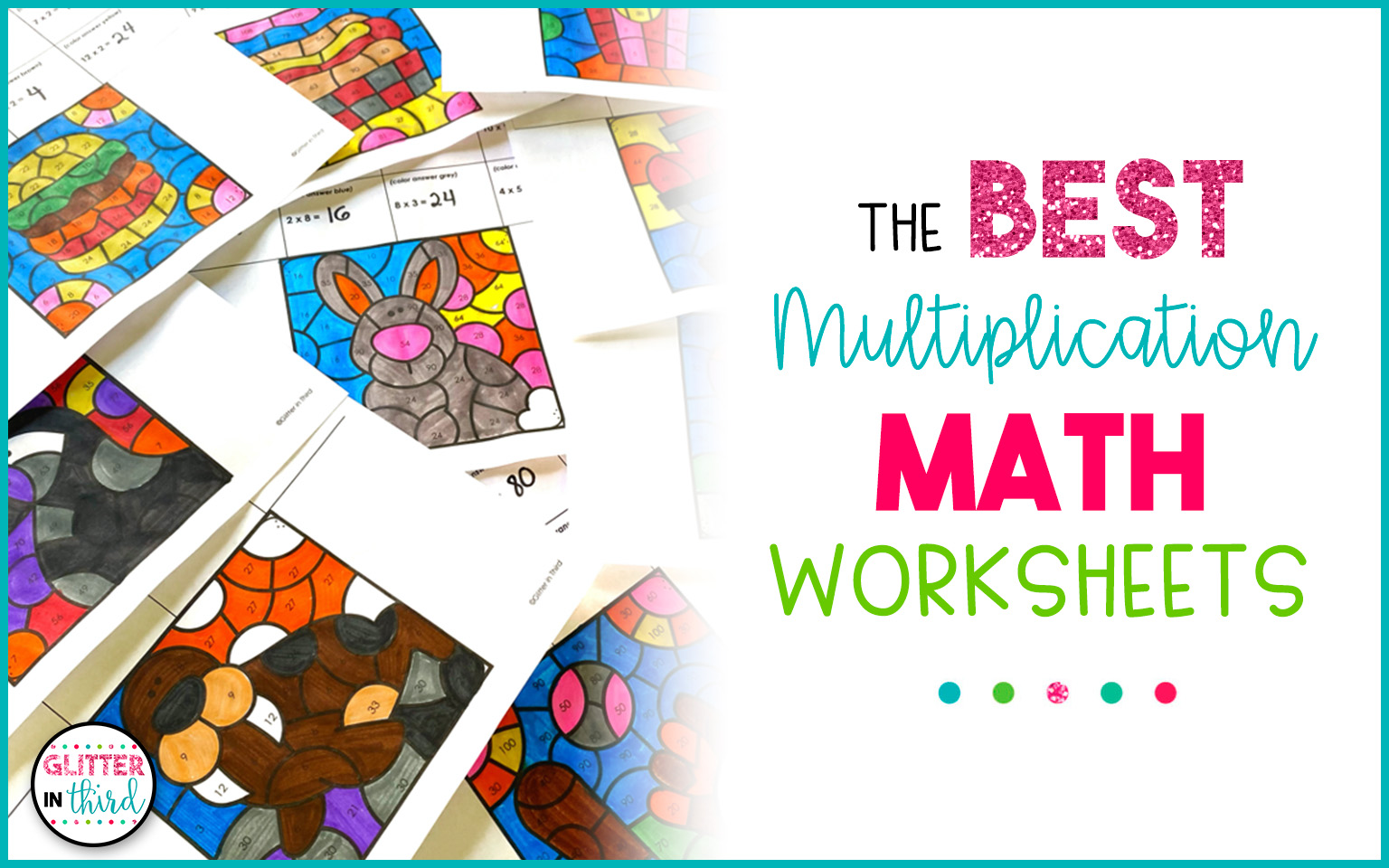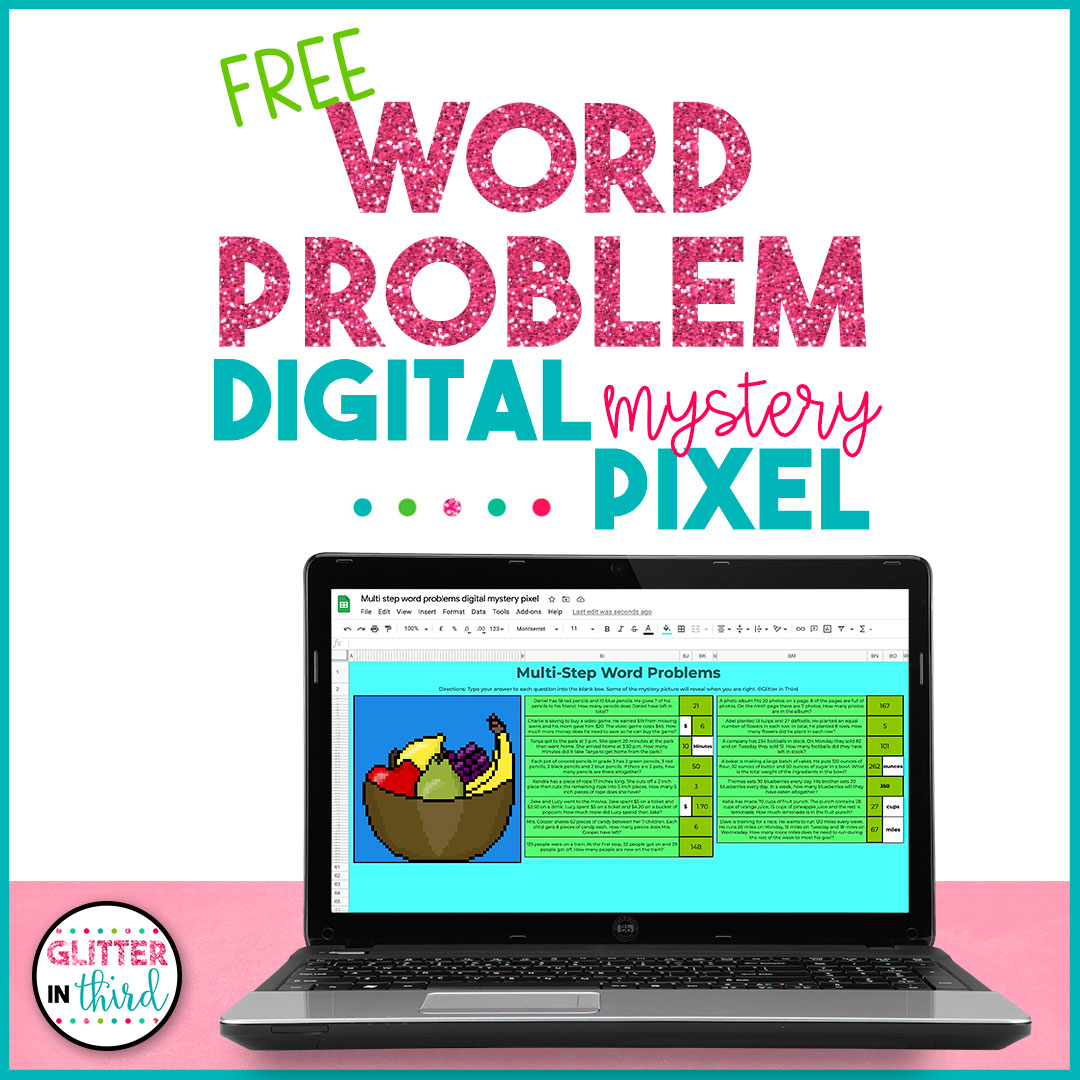# The BEST Multiplication Math WorksheetsLet me tell you about the BEST multiplication math worksheets!

These math facts are made WAY more fun by the way of color-by-numbers!

Multiplication facts can be tedious… but throw in some coloring and suddenly students will WANT to practice their multiplication facts!

## How do students use these multiplication math worksheets?

Let me tell you how these multiplication color by.numbers work!

First, students answer multiplication facts (ranging from 1-12).

Next, students find all the answers on the black-and-white picture at the bottom of the worksheet.

Kids color the picture depending on their multiplication fact answers.

How stinking fun is that?!

## What makes these worksheets so great?

There are three BIG reasons that you will want these multiplication worksheets in your classroom!

First, they are ENGAGING!

Due to the coloring, multiplication facts are much more fun and exciting.

Second, these are NO PREP!

Yep, just print and go. Students do the restFinally, these are MOTIVATING.

Students will want to finish these multiplication worksheets because they will want to see how their picture turns out!

## What math facts are included in these worksheets?

You receive a total of 15 multiplication math worksheets in this set!

Here is a breakdown of types of multiplication questions:

• 10 multiplication worksheets for time tables #1-12 math facts
• 5 mixed multiplication worksheets

You could use these all during your multiplication unit, or use them throughout the year to keep those multiplication math facts fresh in students’ minds.

## Where can I get these multiplication math worksheets?

You can get these at Glitter in Third on TPT.

## Need more multiplication resources?

I have tons more multiplication resources available at Glitter in Third on TPT.

Click the links below to check them out!

Don’t forget to check out my blog post on multiplication picture books – these are THE BEST to use as an introduction to multiplication!## Want FREE math pixel art?

Leave your email and name below to get FREE pixel art for multistep word problems!

As students answer word problems correctly, a mystery picture appears!

The post The BEST Multiplication Math Worksheets appeared first on Glitter in Third.